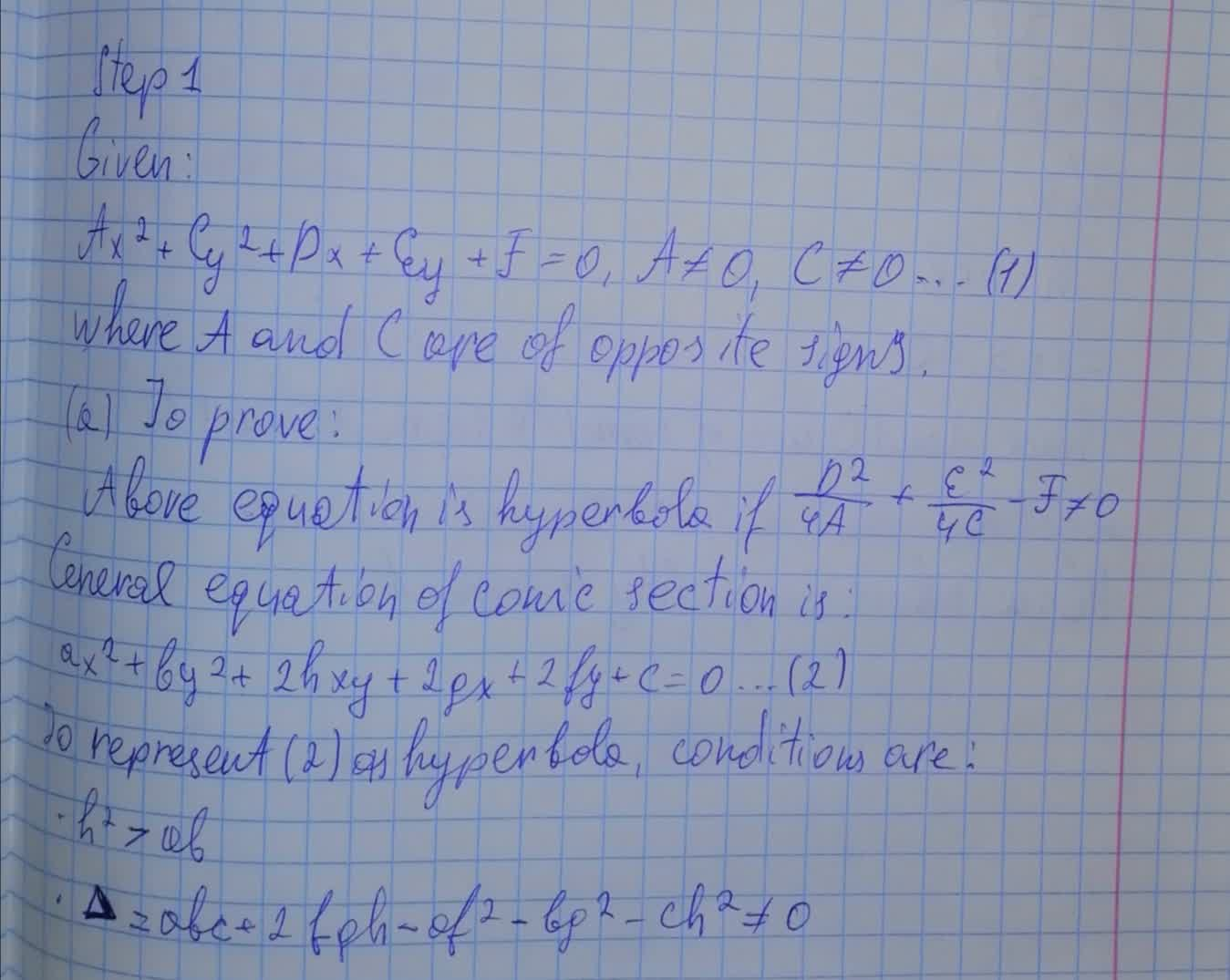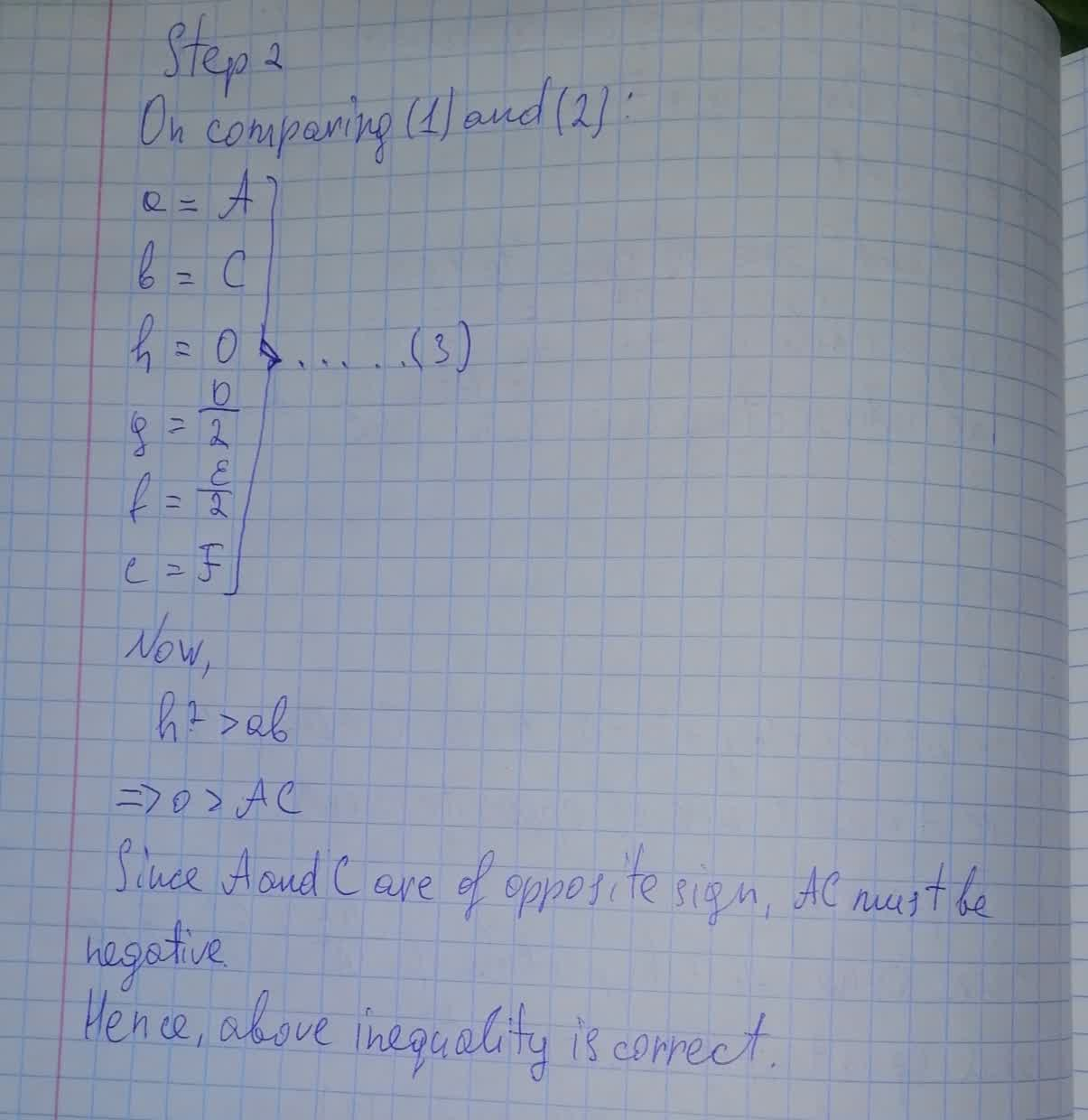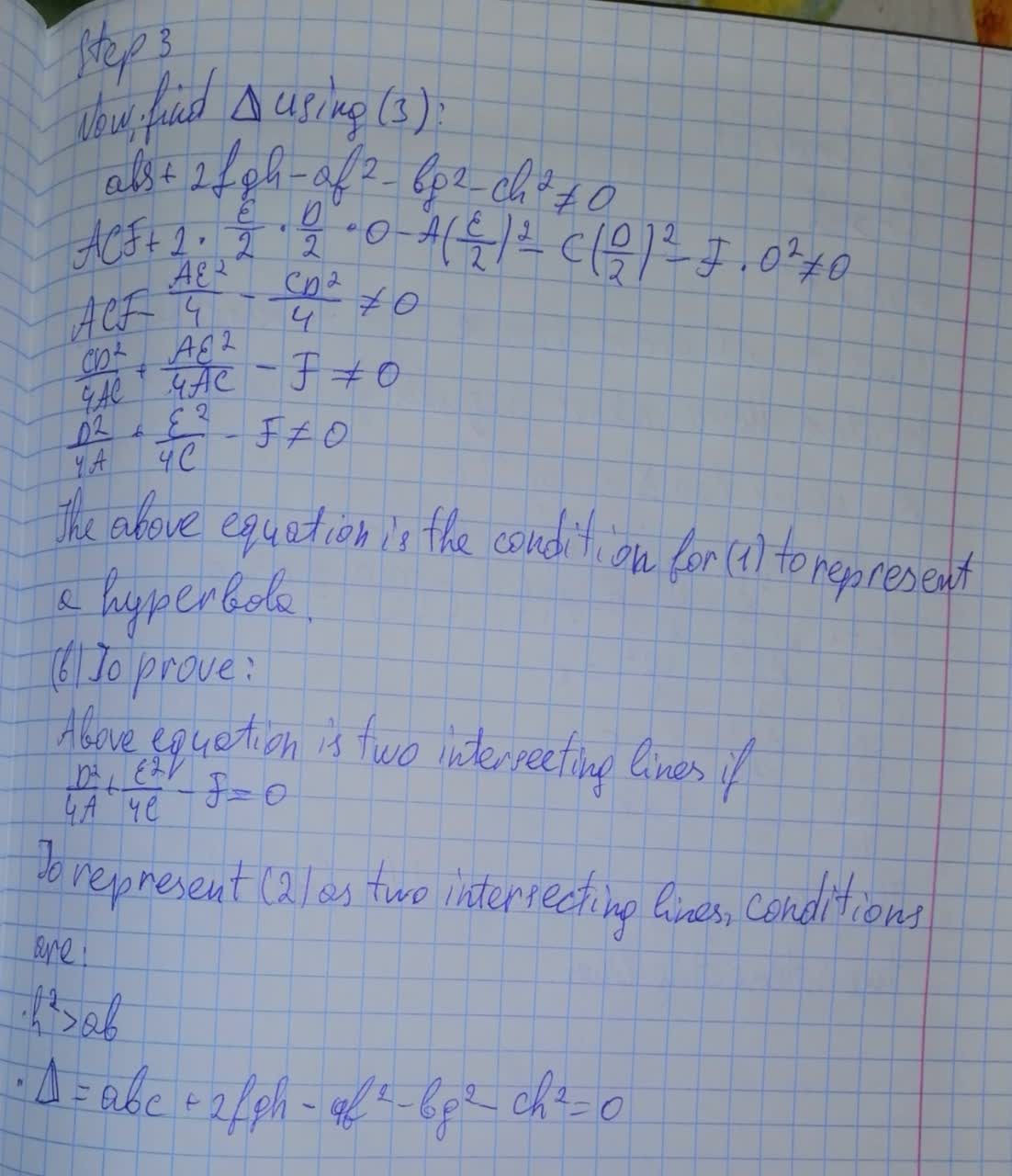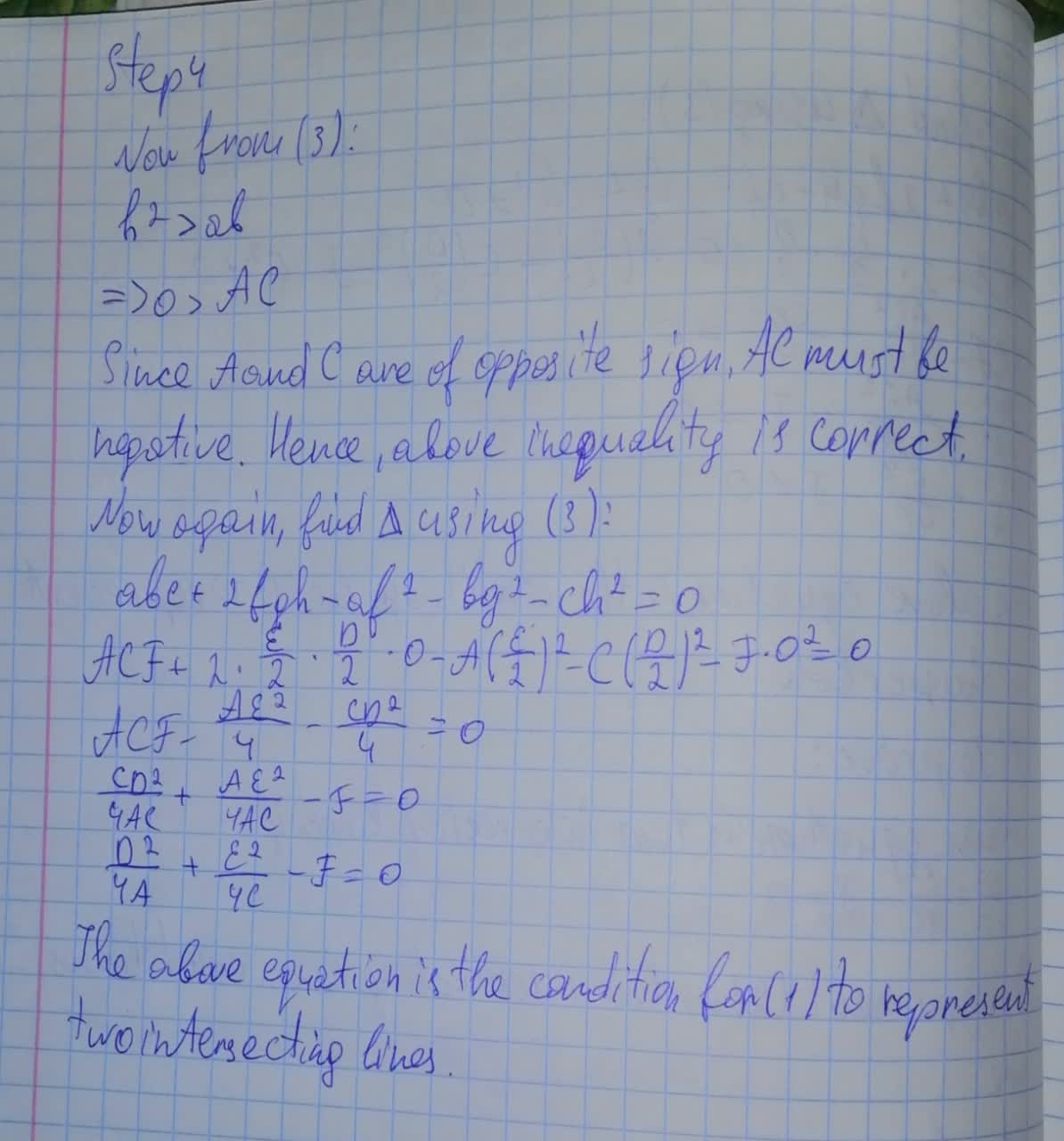# Show that the graph of an equation of the form Ax^2+Cy^2+Dx+Ey+F=0 , A \ne 0 , C \ne 0 , where A and C are of opposite signpancha3 2021-08-10 Answered
Show that the graph of an equation of the form
$$\displaystyle{A}{x}^{{2}}+{C}{y}^{{2}}+{D}{x}+{E}{y}+{F}={0},{A}\ne{0},{C}\ne{0}$$
where A and C are of opposite sign,
(a) is a hyperbola if $$\displaystyle{\frac{{{D}^{{2}}}}{{{4}{A}}}}+{\frac{{{E}^{{2}}}}{{{4}{C}}}}-{F}\ne{0}$$
(b) is two intersecting lines if $$\displaystyle{\frac{{{D}^{{2}}}}{{{4}{A}}}}+{\frac{{{E}^{{2}}}}{{{4}{C}}}}-{F}={0}$$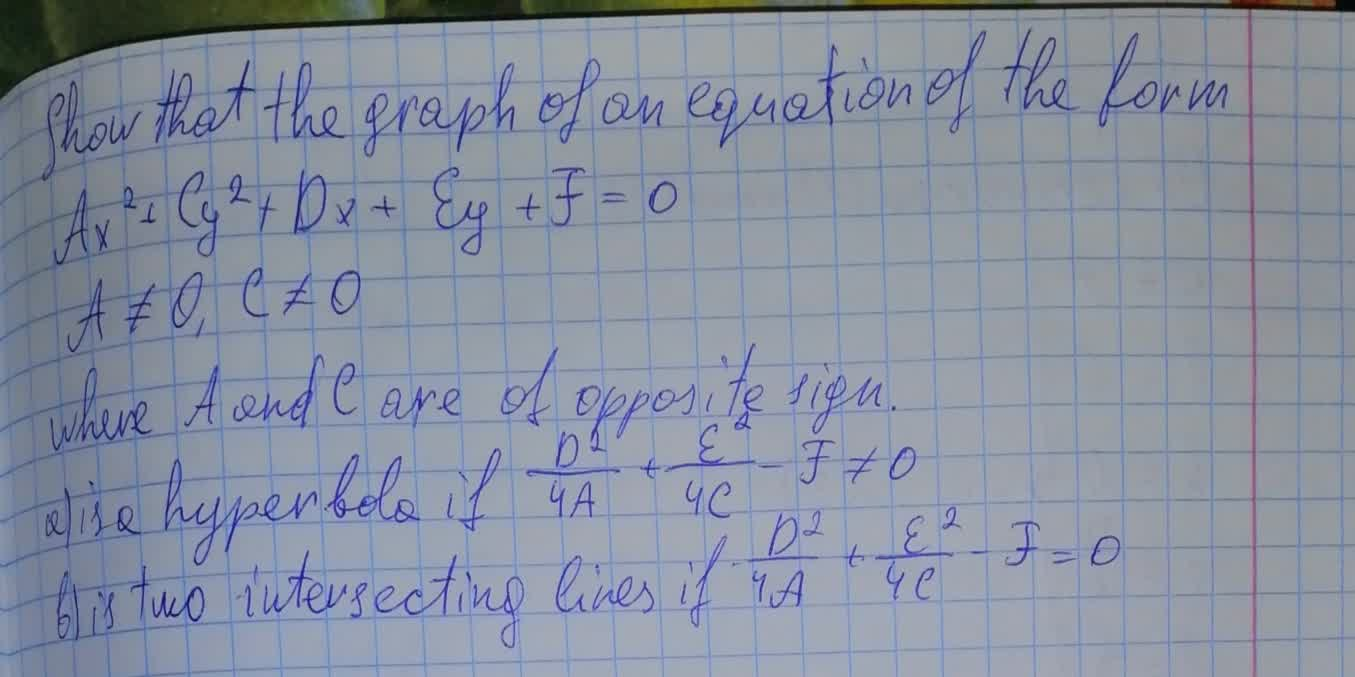• Questions are typically answered in as fast as 30 minutes

### Plainmath recommends

• Get a detailed answer even on the hardest topics.
• Ask an expert for a step-by-step guidance to learn to do it yourself.krolaniaN

Solution below